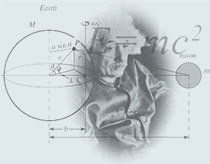Home Page >> TeachingOffice: Old Dominion University Oceanography & Physics Bld. Office: 323 Phone:  (757) 683-5814 Fax:       (757) 683-3038 E-mail:   balitsky@jlab.org Mailing Address: Ian Balitsky Old Dominion University Physics Department 4600 Elkhorn Ave Norfolk, VA 23529
 Physics 425: Electromagnetism I Course Information: Lectures: MW 3:00 - 4:15 p.m. in rm 0204 OCNPS Recitation: W 4:20 - 5:10 p.m. in rm 0204 OCNPS Office hours: M 4:15 - 5:15 p.m. TextBook: D.J. Griffiths, ``Introduction to Electrodynamics’’ 4rd Edition Reference Book: W. Greiner, ``Classical Electrodynamics’’ Course Syllabus:    PDF File Midterm 1 Oct 18, 3 p.m - 5 p.m.. Covered: chapters 2 and 3.    Solutions Midterm 2 Wed Nov 29,, 3:00-5:00 p.m. Covered: chapters 3 and 4. Solutions Final exam (comprehensive): Fri Dec 15, 3:45-6:45 p.m, Room 204. Lecture Notes & Helpful Links: Line integrals    Divergence in spherical coordinates Math Background for Physics 604 Chapter 1    Chapter 2    Chapter 3    Chapter 3 problems    Chapter 4    Chapter 5    Chapter 5 problems    Chapter 6    Chapter 6 problems Magnetic fields far from currents Course Homeworks: HW assignment 1: problem 1.18, problem 1.32, and problem 1.34 form Griffiths (4th ed.).    Solution HW assignment 2: problem 1.41, problem 1.43, and problem 1.49 from Griffiths (4th ed.).    Solution HW assignment 3: problem 2.18, problem 2.20, and problem 2.34 from Griffiths (4th ed.).    Solution HW assignment 4: problem 2.54 except (f) and (g) and problem 3.1 from Griffiths (4th ed.).    Solution HW assignment 5: problems 3.15 and 3.16 from Griffiths (4th ed.).    Solution HW assignment 6: problems 3.24, 3.27, and 3.29 from Griffiths (4th ed.).    Solution HW assignment 7: problems 4.10, 4.19, and 4.20 from Griffiths (4th ed.).    Solution HW assignment 8: problems 4.27 and 4.39 from Griffiths (4th ed.).    Solution HW assignment 9: problems 5.7, 5.11, and 5.13 from Griffiths (4th ed.).    Solution HW assignment 10: problems 5.24, 5.36, and 5.44 from Griffiths (4th ed.).    Solution HW assignment 11: problem 6.17, 6.24, and 6.27 from Griffiths (4th ed.).    Solution.© 2008, ODU Physics Department http://www.balitsky.com